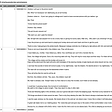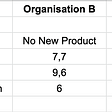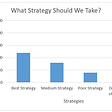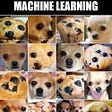# A Crash Course on Hypothesis Testing — A Data Science Approach

Hypothesis testing is a quintessential part of statistical inference in data science context.

In previous article, we talked about the estimating the population parameter such as mean or variance and overview some of the methods to accomplish this goal. The parameter estimation is one of the fundamental components of the statistical inference. The other component is testing hypothesis about those parameters. In this article, I will give you a short introduction on the fundamentals of the hypothesis testing.

# Philosophical Justification

Much of the philosophical justification for the continued use of statistical hypothesis testing seems to be based on the Popper’s proposals for falsification tests of hypothesis. It is stated that “Popper supplied the philosophy and Fisher, Pearson, and colleagues supplied the statistics”.

# Classical Statistical Hypothesis Testing

Classical hypothesis testing rests on two basic concepts. First, a statistical null hypothesis (H0), which is usually (although not necessary) a hypothesis of no difference or no relationship between population parameters, e.g., no difference between the means of two populations. The difference is usually called no effect. So, the null hypothesis is circling around the no effect relationship. This is mainly because the science progress by severely testing and falsifying hypothesis. This is simply indicating that the rejection of hypothesis testing provides a support/corroboration for the alternative or research hypothesis. However, some argues that rejection of the null hypothesis does not provide true corroboration and statistical tests, as currently practiced.

Second, we must choose the test statistic to test the null hypothesis. The test statistic is a random variable and as such, it has a probability distribution. The most common statistic is sampling mean or average of observations. Under null hypothesis, the sampling distribution, represent the probability distribution of test statistic under a repeated sampling from the population.

Basic Hypothesis Testing

The basic proposed hypothesis testing by Sir Ronald Fisher, includes only null hypothesis and consists of the following steps:

• Construct a null hypothesis (H0).
• Choose the test statistic that measure the deviation from the null hypothesis and that has a well-known sampling distribution.
• Collect one or more data from the sampling distribution from the population and compare the value of the test statistic from your sample(s) to its sampling distribution.
• Determine the P-value, which is the probability of obtaining the observed value or one more extreme if H0 is true.
• Reject the H0 if P-value is small and retain if it is large enough.

The P-value is usually stated as the evidence against H0. Fisher also proposed conventional probability for rejecting H0: this is called a significance level. He suggested a probability of one in twenty (0.05 or 5%) as a convenient level and the publication of tables of sampling distributions for various statistics reinforced this by only including tail probabilities beyond these conventional levels (e.g. 0.05, 0.01, 0.001).

Modern Hypothesis Testing

The significance level is interpreted as the proportion of times the H0 would be wrongly rejected using this decision rule if the experiment were repeated many times and the H0 was actually true.

The major difference between the conventional hypothesis testing proposed by Fisher and the modern counterpart proposed by Neyman and Pearson approaches was that Neyman and Pearson explicitly incorporated an alternative hypothesis (HA). The HA is another hypothesis which should be true if the null hypothesis is false. For example, if the H0 is that two population means are equal, then the HA is that they are different by some amount. In contrast, Fisher strongly opposed the idea of HA in significance testing.

The Neyman and Pearson hypothesis testing introduced the notion of type I error, long-run probability of rejecting the H0 when it is actually true and is denoted by alpha, and Type II error, long-run probability of not rejecting Ho when it is actually false which is denoted by beta.

To reiterate, interpretations from classical statistical tests are based on a long-run frequency interpretation of probabilities, i.e. the probability in a long run of identical “trials” or “experiments”. This implies that we have one or more clearly defined population(s) from which we are sampling and for which inferences are to be made. If there is no definable population from which random samples are collected, the inferential statistics discussed here are more difficult to interpret since they are based on long-run frequencies of occurrence from repeated sampling. Randomization tests (Section 3.3.2), which do not require random sampling from a population, may be more applicable.

# Associated probability and Type I error

The P value can be expressed as P(data|H0), the probability of observing our sample data, or data more extreme, under repeated identical experiments if the H0 is true. This is not the same as the probability of H0 being true, given the observed data — P(H0|data). If we wish to know the probability of H0 being true, we need to tackle hypothesis testing from a Bayesian perspective

# Hypothesis Tests for a Single Population

The single population test is about the testing hypothesis about single population parameters or about the difference between two population parameters if certain assumption about the variable hold. Sometimes testing an H0 that the mean equals zero is relevant, e.g., the mean change from before to after a treatment equals zero, and testing whether other parameters equal zero. To this end, we usually use the t-statistic which the general form is:

where St is the value of the statistics from our sample, \theta is the population value against which the sample statistic is to be tested as specified in H0 and S_st is the estimated standard error of the sample statistic. Here is a simple example:

• Specify the H0 (e.g., mean=0) and HA (e.g., mean not equal 0)
• Take a random sample from clearly defined population
• Calculate t = (y-0)/sy from the sample, where the sy is the estimated standard error of the sample mean. Note that if H0 is true, we would expect t to be close to zero, i.e. when we sample from a population with a mean of zero, most samples will have means close to zero. Sample means further from zero are less likely to occur if H0 is true.
• Compare t with the sampling distribution of t at 0.05 (or 0.01 or whatever significance level you choose a priori) with n-1 df. This value of t is sometimes called the critical value. If the probability (P value) of obtaining our sample t value or one larger is less than 0.05 (our ), then we reject the H0.

If we compare our t-statistic with the critical value at both end of the sampling distribution, then it is called two-tail tests otherwise it is called one-tail test.

The hypothesis tests for the single value is equivalent to the checking that if the confidence interval (with a given significance level) overlaps with zero value.

# Hypothesis Tests for Two Populations

There are tests about the equivalent parameter in two population. If we have two random sample from each of two independent population, i.e., the population represent different collection of observation. For example, to the H0 that mean1=mean2 (comparing two independent population means):

where the mixed standard deviation is

If we have two set of observation paired with each other (paired samples), then we actually deal with the single hypothesis testing.

# Critical Assumptions

All statistical tests have some assumptions and if these assumptions are not met then the hypothesis testing is not reliable.

The first assumption is that the samples are from normally distributed populations. However, there is reasonable evidence from simulation studies that significance tests based on the t test are usually robust to violations of this assumption unless the distributions are very non-symmetrical, e.g. skewed or multi- modal. Transformations of the variable to a different scale of measurement can often improve its normality.

The second assumption is that the samples are from population with equal variance. Again, the evidence shows that the t test is robust enough to violation of this assumption.

Statistical hypothesis testing should be used carefully, preferably in situations where power and effect sizes have been considered

# Randomization (Permutation) Tests

These tests resample and reshuffle the original data many times to generate the sampling distribution of a test statistic directly. The general steps in conducting a randomization test are

• Calculate the difference between the means of two samples
• Randomly draw n1 number of observations and label them as samples 1 and the rest as the samples 2.
• Repeat the second step a large number of times, each time calculate the mean differences, call them Di.
• Calculate the proportion of all the Dis that are greater than or equal to D0 (the difference between the means in our samples). This is the “P value” and it can be compared to an a priori significance level (e.g. 0.05) to decide whether to reject the H0 or not (Neyman–Pearson tradition), or used as a measure of “strength of evidence” against the H0.

The underlying principle behind randomization tests is that if the null hypothesis is true, then any random arrangement of observations to groups is equally possible.

# Multiple Testing

In the multiple testing, as its name, there are multiple comparisons. The problem with multiple testing is that, as the number of tests increases, the probability that at least one Type I error among the collection of tests increases as well. In the case of independent multiple testing, the probability of at least Type I error is given as follows:

where alpha is the significance level (e.g., 0.05) for each test and c is the number of tests. In the table below, the one Type I probability is shown.

In other words, if you keep asking different questions (multiple hypothesis testing), there is more chance to draw a conclusion that the pattern exists which might happen by chance!

There are some approaches recommended for controlling the inflammatory alpha. Some proposed solutions are Bonferroni procedure, Dun-Sidak procedure, and sequential Bonferroni.

# Critique of Statistical Hypothesis Testing

The statistical hypothesis testing is criticized by many statisticians. The first and the main limitation of the hypothesis testing is that it depends on the sample size, i.e., everything else being the same, larger sample sizes are more likely to produce a statistically significant result and with very large sample sizes, trivial effects can produce a significant result. For this reason, designing experiments based on a priori power considerations is crucial here. Rather than arbitrarily choosing sample sizes, our sample size should be based on that necessary to detect a desired effect if it occurs in the population(s). Keep in mind that, interpreting significance tests should always be done in conjunction with a measure of effect size (e.g. difference between means) and some form of confidence interval.

--

--

--

## More from Analytics Vidhya

Analytics Vidhya is a community of Analytics and Data Science professionals. We are building the next-gen data science ecosystem https://www.analyticsvidhya.com

## Data visualisation begins in your mind## Cognitive AI, ML, and Recommendations — Saxon## How to Collect a Reddit Dataset## Scikit-Learn for Text Analysis of Amazon Fine Food Reviews## Data is the new fuel## Flipping the Script from Reactive to Proactive with Prescriptive Analytics (Part 4)## Maximin Strategy in Game Theory## The Data Science Process## Vahid Naghshin

Technology enthusiast, Futuristic, Telecommunications, Machine learning and AI savvy, work at Dolby Inc.

## What Actually Makes a Good Category?## Hypothesis, Parametric and Non-Parametric Testing## Solving a machine learning problem never means jumping right of from the data and starting…## 6 key mistakes junior (and not so junior) Data Scientists make